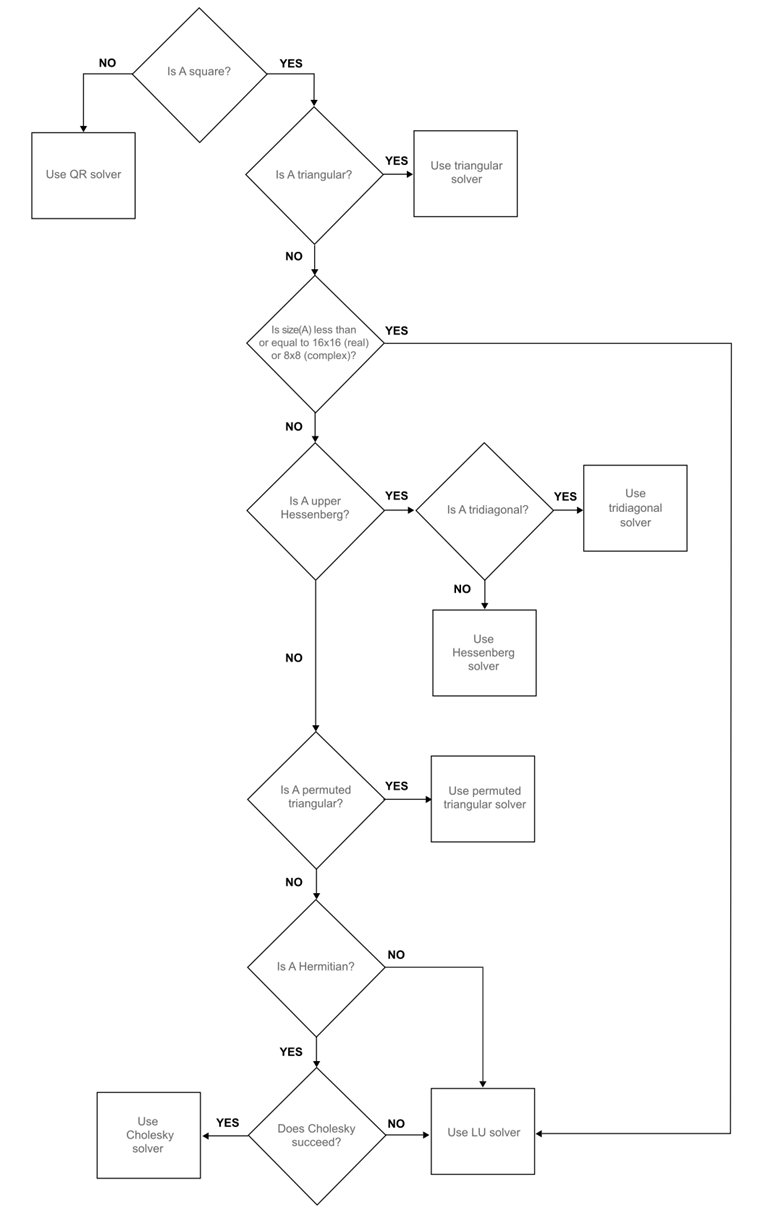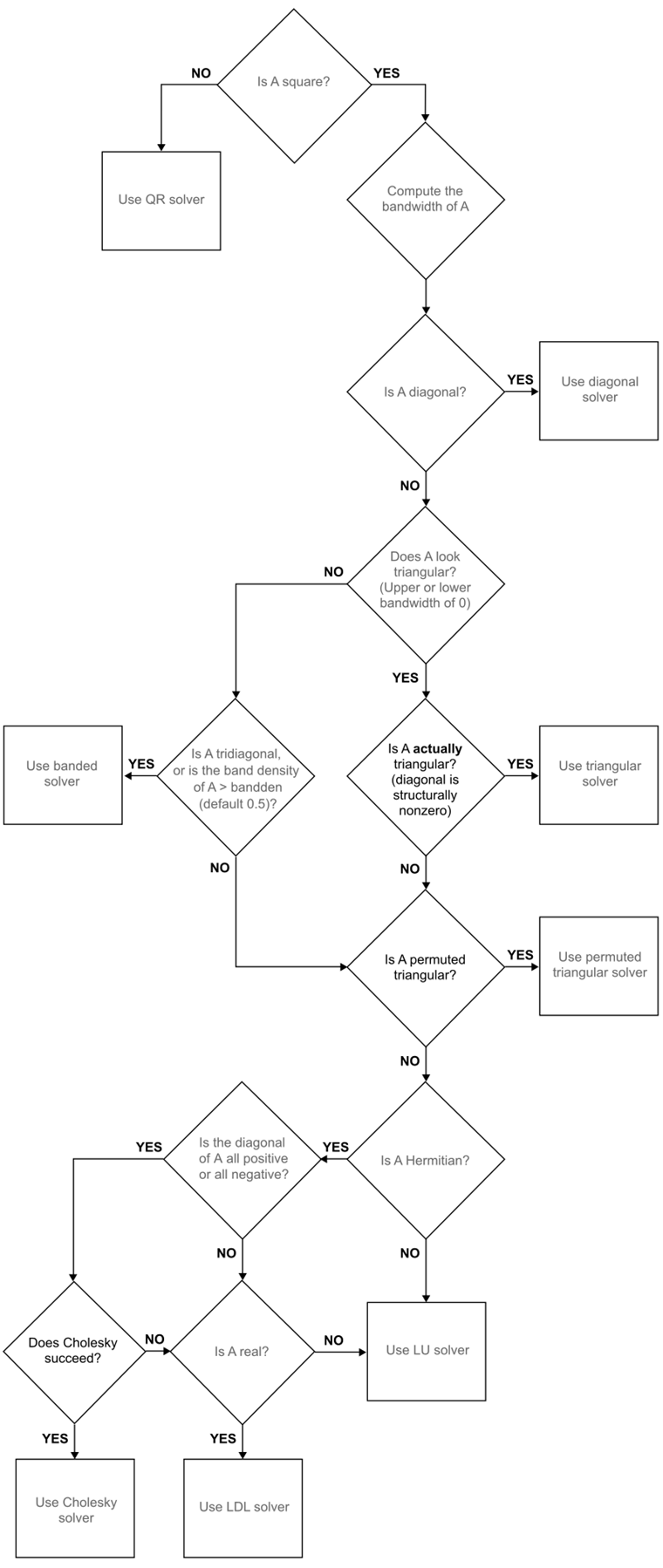# mldivide, \

## 语法

``x = A\B``
``x = mldivide(A,B)``

## 说明

``x = A\B` 对线性方程组 `A*x = B` 求解。矩阵 `A` 和 `B` 必须具有相同的行数。如果 `A` 未正确缩放或接近奇异值，MATLAB® 将会显示警告信息，但还是会执行计算。如果 `A` 是标量，那么 `A\B` 等于 `A.\B`。如果 `A` 是 `n`×`n` 方阵，`B` 是 `n` 行矩阵，那么 `x = A\B` 是方程 `A*x = B` 的解（如果存在解的话）。如果 `A` 是矩形 `m`×`n` 矩阵，且 `m ~= n`，`B` 是 `m` 行矩阵，那么 `A`\`B` 返回方程组 `A*x= B` 的最小二乘解。`
` `x = mldivide(A,B)` 是执行 `x` `=` `A`\`B` 这一操作的替代方法，但很少使用。它可以启用类的运算符重载。`

## 示例

```A = magic(3); B = [15; 15; 15]; x = A\B```
```x = 3×1 1.0000 1.0000 1.0000 ```

```A = magic(4); b = [34; 34; 34; 34]; x = A\b```
```Warning: Matrix is close to singular or badly scaled. Results may be inaccurate. RCOND = 1.306145e-17. ```
```x = 4×1 1.5000 2.5000 -0.5000 0.5000 ```

`rcond` 等于 `0` 时，会出现奇异警告。

```A = [1 0; 0 0]; b = [1; 1]; x = A\b```
```Warning: Matrix is singular to working precision. ```
```x = 2×1 1 Inf ```

```A = [1 2 0; 0 4 3]; b = [8; 18]; x = A\b```
```x = 3×1 0 4.0000 0.6667 ```

```A = sparse([0 2 0 1 0; 4 -1 -1 0 0; 0 0 0 3 -6; -2 0 0 0 2; 0 0 4 2 0]); B = sparse([8; -1; -18; 8; 20]); x = A\B```
```x = (1,1) 1.0000 (2,1) 2.0000 (3,1) 3.0000 (4,1) 4.0000 (5,1) 5.0000 ```

## 输入参数

• 如果 `A``B` 具有整数数据类型，则另一个输入必须为标量。整数数据类型的操作数不能为复数。

## 提示

• 运算符 `/``\` 通过以下对应关系而相互关联：`B/A = (A'\B')'`

• 如果 `A` 是方阵，则 `A\B` 约等于 `inv(A)*B`，但 MATLAB 会用不同的更为稳健的方式处理 `A\B`，而且效果更好。

• 如果 `A` 的秩小于 `A` 中的列数，则 `x = A\B` 不一定是最小范数解。您可以使用 `x = lsqminnorm(A,B)``x = pinv(A)*B` 来计算最小范数最小二乘解。

• 使用 `decomposition` 对象多次高效地求解具有不同右侧的线性方程组。`decomposition` 对象非常适合求解需要重复求解的问题，因为系数矩阵的分解不需要执行多次。

## 算法

`mldivide` 在求解线性方程组方面的多用性源于其能够在调度适当的求解器时利用问题中的对称性。此方法旨在最大限度减少计算时间。该函数首先区分（也称为“稠密”）和稀疏输入数组。

### 针对完全输入的算法### 针对稀疏输入的算法Gilbert, John R., and Tim Peierls. “Sparse Partial Pivoting in Time Proportional to Arithmetic Operations.” SIAM Journal on Scientific and Statistical Computing 9, no. 5 (September 1988): 862–874. https://doi.org/10.1137/0909058.

 Anderson, E., ed. LAPACK Users’ Guide. 3rd ed. Software, Environments, Tools. Philadelphia: Society for Industrial and Applied Mathematics, 1999. https://doi.org/10.1137/1.9780898719604.

 Davis, Timothy A. "Algorithm 832: UMFPACK V4.3 – an unsymmetric-pattern multifrontal method." ACM Transactions on Mathematical Software 30, no. 2 (June 2004): 196–199. https://doi.org/10.1145/992200.992206.

 Duff, Iain S. “MA57---a Code for the Solution of Sparse Symmetric Definite and Indefinite Systems.” ACM Transactions on Mathematical Software 30, no. 2 (June 2004): 118–144. https://doi.org/10.1145/992200.992202.

 Davis, Timothy A., John R. Gilbert, Stefan I. Larimore, and Esmond G. Ng. “Algorithm 836: COLAMD, a Column Approximate Minimum Degree Ordering Algorithm.” ACM Transactions on Mathematical Software 30, no. 3 (September 2004): 377–380. https://doi.org/10.1145/1024074.1024080.

 Amestoy, Patrick R., Timothy A. Davis, and Iain S. Duff. “Algorithm 837: AMD, an Approximate Minimum Degree Ordering Algorithm.” ACM Transactions on Mathematical Software 30, no. 3 (September 2004): 381–388. https://doi.org/10.1145/1024074.1024081.

 Chen, Yanqing, Timothy A. Davis, William W. Hager, and Sivasankaran Rajamanickam. “Algorithm 887: CHOLMOD, Supernodal Sparse Cholesky Factorization and Update/Downdate.” ACM Transactions on Mathematical Software 35, no. 3 (October 2008): 1–14. https://doi.org/10.1145/1391989.1391995.

 Davis, Timothy A. “Algorithm 915, SuiteSparseQR: Multifrontal Multithreaded Rank-Revealing Sparse QR Factorization.” ACM Transactions on Mathematical Software 38, no. 1 (November 2011): 1–22. https://doi.org/10.1145/2049662.2049670.